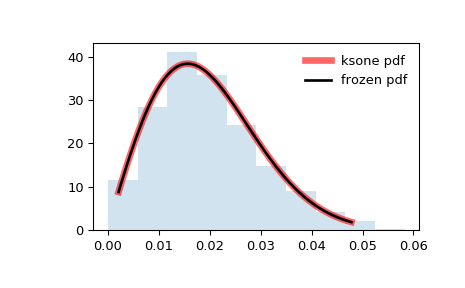# scipy.stats.ksone¶

scipy.stats.ksone = <scipy.stats._continuous_distns.ksone_gen object>[source]

General Kolmogorov-Smirnov one-sided test.

As an instance of the rv_continuous class, ksone object inherits from it a collection of generic methods (see below for the full list), and completes them with details specific for this particular distribution.

Examples

>>> from scipy.stats import ksone
>>> import matplotlib.pyplot as plt
>>> fig, ax = plt.subplots(1, 1)


Calculate a few first moments:

>>> n = 1e+03
>>> mean, var, skew, kurt = ksone.stats(n, moments='mvsk')


Display the probability density function (pdf):

>>> x = np.linspace(ksone.ppf(0.01, n),
...                 ksone.ppf(0.99, n), 100)
>>> ax.plot(x, ksone.pdf(x, n),
...        'r-', lw=5, alpha=0.6, label='ksone pdf')


Alternatively, the distribution object can be called (as a function) to fix the shape, location and scale parameters. This returns a “frozen” RV object holding the given parameters fixed.

Freeze the distribution and display the frozen pdf:

>>> rv = ksone(n)
>>> ax.plot(x, rv.pdf(x), 'k-', lw=2, label='frozen pdf')


Check accuracy of cdf and ppf:

>>> vals = ksone.ppf([0.001, 0.5, 0.999], n)
>>> np.allclose([0.001, 0.5, 0.999], ksone.cdf(vals, n))
True


Generate random numbers:

>>> r = ksone.rvs(n, size=1000)


And compare the histogram:

>>> ax.hist(r, normed=True, histtype='stepfilled', alpha=0.2)
>>> ax.legend(loc='best', frameon=False)
>>> plt.show()Methods

 rvs(n, loc=0, scale=1, size=1, random_state=None) Random variates. pdf(x, n, loc=0, scale=1) Probability density function. logpdf(x, n, loc=0, scale=1) Log of the probability density function. cdf(x, n, loc=0, scale=1) Cumulative distribution function. logcdf(x, n, loc=0, scale=1) Log of the cumulative distribution function. sf(x, n, loc=0, scale=1) Survival function (also defined as 1 - cdf, but sf is sometimes more accurate). logsf(x, n, loc=0, scale=1) Log of the survival function. ppf(q, n, loc=0, scale=1) Percent point function (inverse of cdf — percentiles). isf(q, n, loc=0, scale=1) Inverse survival function (inverse of sf). moment(n, n, loc=0, scale=1) Non-central moment of order n stats(n, loc=0, scale=1, moments='mv') Mean(‘m’), variance(‘v’), skew(‘s’), and/or kurtosis(‘k’). entropy(n, loc=0, scale=1) (Differential) entropy of the RV. fit(data, n, loc=0, scale=1) Parameter estimates for generic data. expect(func, args=(n,), loc=0, scale=1, lb=None, ub=None, conditional=False, **kwds) Expected value of a function (of one argument) with respect to the distribution. median(n, loc=0, scale=1) Median of the distribution. mean(n, loc=0, scale=1) Mean of the distribution. var(n, loc=0, scale=1) Variance of the distribution. std(n, loc=0, scale=1) Standard deviation of the distribution. interval(alpha, n, loc=0, scale=1) Endpoints of the range that contains alpha percent of the distribution